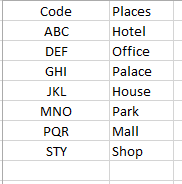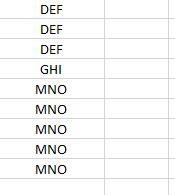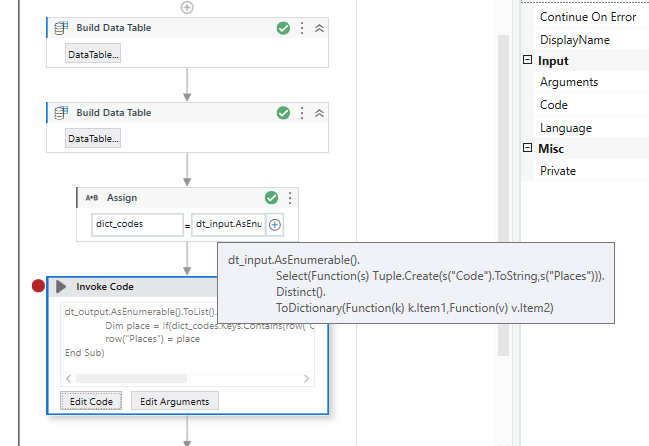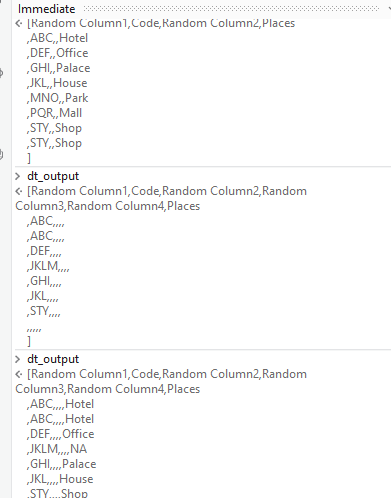# LINQ Query for matching row from another Excel column and update the Result

Linq Query having two Datatables, if code matches then need to update the result in another column.1 Like

Hi Shubham,

hope this helps.
matching.xaml (10.0 KB)
Dummy.xlsx (13.4 KB)

@Dmitri00007 , thanks but result should be if Code DEF equal to DEF from another workbook, then result would be Office

Have you tried using the `Join Datatables` Activity with `Left Join` as the Join Type ? Keep the Datatable whose column needs to be updated as the Datatable 1 and the other Datatable as Datatable 2. You should be able to receive the required Output.

We can then use `Filter Datatable` Activity and preserve the columns which are required in the Output.

Let us know if you are still unable to follow the Steps.

Thanks @supermanPunch , I had multiple columns and if I use JoinDatatable Activity further it will create more complexity too solve the real Scenario. so Looking for LINQ.

Could you let us know if you have to join with Multiple Columns or Do you just have multiple columns in the Datatable/Excel Data and would want to preserve only the Required Columns at last as the Output. Even for this Scenario, we would go for the `Join Datatables` Activity at first, if the result is not as expected, we then try with the Linq approach.

For the case similar to as described , we go with activities that are available and check the outcome for different data that is present.

Let us know if you are still looking for a Linq approach.

@supermanPunch Yes buddy looking for LINQ

Since you have only mentioned that there are extra columns, I’ll be making a couple of assumptions here:

• Both, input and output datatables have numerous columns, but only Code and Places are taken into consideration from input
• The output data only requires Places(assumption, since the column name was not provided in the second screenshot) to be updated

If the assumptions provided about are accurate, then could you give this workflow a try?``````//Create a Dictionary to create convert Code, Palace into Key-Value pairs
dt_input.AsEnumerable().
Select(Function(s) Tuple.Create(s("Code").ToString,s("Places"))).
Distinct().
ToDictionary(Function(k) k.Item1,Function(v) v.Item2)
``````
``````//Use an Invoke Code to update column in output Datatable
dt_output.AsEnumerable().ToList().ForEach(Sub(row)
Dim place = If(dict_codes.Keys.Contains(row("Code").ToString),dict_codes(row("Code").ToString),"NA")
row("Places") = place
End Sub)
``````UpdateDatainSecondDataTable.xaml (13.3 KB)

Kind Regards,
Ashwin A.K

1 Like

@ashwin.ashok buddy in regards, first LINQ that created
dict_codes dictionary(String,Object) = dt_config.AsEnumerable().
Select(Function(s) Tuple.Create(s(“Code”).ToString,s(“Places”))).
Distinct().
ToDictionary(Function(k) k.Item1,Function(v) v.Item2)
Showing an error